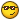# Decimal To Fraction

## Recommended Posts

I have created a function that takes a non-integer (float) and turns it into a fraction. If anyone is interested then check out this code:

```; Useage - D2F( decimal number )
; Return - one dimensional array with two elements:
; element = numerator
; element = denominator
; On failure it will set @error to 1 and return a blank string.

Func D2F( \$dec )
If IsInt(\$dec) Then
SetError(1)
Return ""
EndIf
Local \$i = 1, \$bNeg = (\$dec < 0), \$f
If \$bNeg Then \$dec *= -1
Do
\$i += 1
\$f = \$dec * \$i
Until IsInt(\$f)
If \$bNeg Then \$f *= -1
\$f = \$i
Return \$f
EndFunc```
It can also simplify a fraction, as shown in this example:
```\$n = 28
\$d = 44

\$f = \$n / \$d
\$a = D2F(\$f)

If @error Then Exit
MsgBox(0, \$f, \$a & @CRLF & \$a); Would return 7/11 instead of 28/44```

Any comments/questions are greatly appreciated!Edited by erifash
##### Share on other sites

I have created a function that takes a non-integer (float) and turns it into a fraction. If anyone is interested then check out this code:

```; Useage - D2F( decimal number )
; Return - one dimensional array with two elements:
; element = numerator
; element = denominator
; On failure it will set @error to 1 and return a blank string.

Func D2F( \$dec )
If IsInt(\$dec) Then
SetError(1)
Return ""
EndIf
Local \$i = 1, \$bNeg = (\$dec < 0), \$f
If \$bNeg Then \$dec *= -1
Do
\$i += 1
\$f = \$dec * \$i
Until IsInt(\$f)
If \$bNeg Then \$f *= -1
\$f = \$i
Return \$f
EndFunc```
It can also simplify a fraction, as shown in this example:
```\$n = 28
\$d = 44

\$f = \$n / \$d
\$a = D2F(\$f)

If @error Then Exit
MsgBox(0, \$f, \$a & @CRLF & \$a)```

Any comments/questions are greatly appreciated!This is great. It could be very usefull for some one who deals with large floats in their script. Just out of curiousity did you just do this for the fun on it or is it part of a larger project? I feel like most people will not see the greatness of your function but to some one who needs it, it will be a treasured.

##### Share on other sites

Thanks! Yeah, it is just a little thing I wanted to do. My calculator (TI-83+) has a function to convert a decimal to a fraction so I thought, "If a simple calculator can do it, why can't AutoIt?"## Create an account

Register a new account

• ### Recently Browsing   0 members

×

• Wiki

• Back

• #### Beta

• Git
• FAQ
• Our Picks
×
• Create New...